# Low-overhead quantum computing with Gottesman-Kitaev-Preskill qubits

by Kyungjoo Noh and Christopher Chamberland | on | in | Permalink |  Share

## Introduction

This post summarizes a research paper from the AWS Center for Quantum Computing that proposes a direction to implement fault-tolerant quantum computers with minimal hardware overhead. This research shows that by concatenating the surface code with Gottesman, Kitaev, and Preskill (GKP) qubits, it is theoretically possible to achieve a logical error rate of 10-8 that is much lower than error rates of current state-of-the-art hardware.

Quantum computers hold the promise of being able to solve certain families of problems exponentially faster than the best classical computers we can hope to build. Such problems include simulating the dynamics of molecules, which has many industrial applications. However, one of the biggest challenges in building quantum computers is that the qubits used to store the quantum information, and the gates used to manipulate the state of the qubits, are sensitive to noise. Noise, such as undesired interactions with the environment surrounding a quantum computer, can lead to errors in the system, thus corrupting the result of the computation.

The quantum computing industry is working to engineer better quantum hardware components, which reduce the likelihood of errors during a computation. Most known quantum algorithms with a computational advantage require very low error rates. Depending on the size of the algorithm, required error rates can range between 10−6 and 10−20. Without error correction, such low error rates are unlikely to be achieved with current approaches to building quantum hardware. The best-known way to achieve lower error rates is to encode the physical qubits and gates in an error-correcting code. The act of encoding uses several physical qubits for each error-corrected logical qubit of an algorithm. Such redundant qubits are used to detect and correct errors whenever they occur. Detecting errors requires the implementation of gates between the physical qubits and must be done in a fault-tolerant way to ensure that correctable errors don’t spread to become large uncorrectable errors (that is, an error which can no longer be fixed by the error-correcting code). The extra redundancy of error correction and fault-tolerance can add significant resource costs for implementing a given algorithm. At the AWS Center for Quantum Computing, we are researching designs of fault-tolerant quantum computers using the minimal resource requirements for hardware architectures.

In this post and the underlying paper, we dive deep into how the GKP qubits work and how they can be more advantageous than two-level qubits. We also explain some key concepts of quantum error correction and fault tolerance. This blog post is intended for a technical audience with an undergraduate level understanding of quantum mechanics.

## Quantum error correction and fault tolerance

An important concept in error correction is the notion of a code distance and threshold. In what follows, for square patch surface codes, the distance d of the code corresponds to the number of data qubits along the horizontal or vertical direction of the lattice. The number of correctable errors is half the code distance, and thus more errors can be corrected by increasing the distance d. For a given fault-tolerant implementation of an error-correcting code, a threshold is an error rate below which, if physical qubits and gates fail with a probability less than the threshold, error correction will exponentially reduce the overall failure probability of the quantum computer. Above the threshold, error correction does more harm than good, as you can see in Figure 1. In order to implement a fault-tolerant error-correcting code with low hardware requirements, the physical error rates of the hardware (qubits and gates) must typically be at least an order of magnitude below the threshold of an error-correcting code. Otherwise, building larger error-correcting codes with more hardware will only modestly improve the failure probability of the encoded qubits and gates (the logical error rate). Obtaining low physical hardware error rates is incredibly challenging. It is imperative to use error-correcting codes with high thresholds. For practical quantum computing with low hardware overhead, you must use error-correcting codes with small footprints and low logical error rates when the physical error rate is well below threshold. These requirements pose a challenge for most suitable error correction candidates.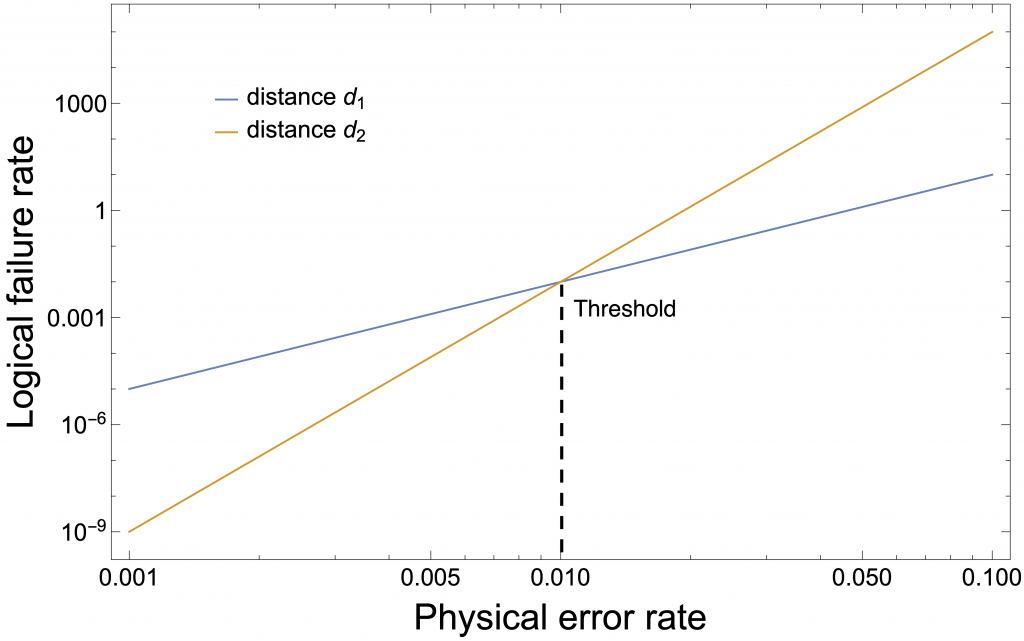Figure 1: Visualization of the threshold error rate. This graph shows an example of two logical error rate curves of an error-correcting code with distances labelled d1 and d2. The distances are related to the size of the code (a larger code corrects more errors), and d1 < d2. In this example, the two curves intersect at the threshold of the code which is 1%. Below the threshold, the larger code has a smaller logical failure rate, but does more harm than good above the threshold.

Given the hardware constraints of superconducting qubit architectures, where gates between qubits can only be implemented via nearest neighbor interactions, topological quantum error-correcting codes are the most promising families of error-correcting codes to be realized on near-term devices. This is because all operations required to detect errors with such codes respect the hardware constraints. Among the known error-correcting codes belonging to the topological code family, surface codes appear to be the most promising candidates.

Surface codes have several desired properties such as high thresholds (about 1% for a toy depolarizing noise model) and a small qubit footprint for a given code distance. For a square surface code of distance d, the total number of qubits is 2d2-1. Furthermore, surface codes require a small number of operations to detect errors relative to other topological error-correcting codes (in more technical terms, the surface code’s stabilizer generators have weight at most four). Most known codes from topological code families, which are not surface codes (which we refer to as alternative topological codes) have lower thresholds and require more operations to detect errors. Consequently, the logical error rates of alternative topological codes below threshold are much higher than that of the surface code, resulting in a much larger resource costs for implementing algorithms.

Various types of qubits can be used to construct the surface code. A popular choice is to use two-level qubits such as superconducting qubits or trapped-ion qubits. However in recent years, new types of qubits have been proposed and experimentally realized. Notable examples include bosonic qubits such as cat qubits and GKP qubits. Bosonic qubits are different from two-level qubits because information is encoded in a simple harmonic oscillator mode, such as a resonant inductor-capacitor circuit, which has infinitely many energy levels. Because information can be redundantly encoded in many more levels, bosonic qubits are themselves protected via bosonic quantum error correction. Now, let’s focus on GKP qubits and go over their unique advantages.

## Bosonic GKP qubits: circumventing Heisenberg’s uncertainty principle

We recently studied the surface-GKP code, which is the surface code consisting of bosonic GKP qubits as opposed to two-level qubits. A bosonic qubit is a qubit encoded in an oscillator mode (such as a microwave resonator) [Gottesman et al., PRA 64, 012310 (2001)]. The upshot of our research is that we can significantly reduce the resource overhead for achieving the same target logical error rate with GKP qubits. This is possible because GKP qubits are themselves error corrected, and the analog information obtained from the GKP error correction can significantly boost the performance of the outer code, i.e., the surface code.

The GKP qubit is named after its inventors Gottesman, Kitaev, and Preskill, and is an example of a bosonic qubit. The key idea behind the GKP qubit is to work around the Heisenberg uncertainty principle. According to Heisenberg’s uncertainty principle, it is impossible to measure both the position and momentum of a particle precisely and simultaneously. This is why even a vacuum state, an oscillator without any photons, has non-zero noise variances in the position and momentum axes (see the Gaussian noise profile in Figure 2a). One way to work around the uncertainty principle is to give up the simultaneity. More accurately, you can reduce the noise variance in the position axis at the expense of an increased noise variance in the momentum axis, hence squeezing the noise (compare Figure 2a with Figure 2b). Note that the extent to which a state is squeezed is conventionally measured in the unit decibel (or dB) due to its close relation to amplification. Compare Figures 2b and 2c to get a sense of what the squeezed states look like at two different squeezing levels (10 dB and 12 dB). So, if you give up measuring the momentum, you can measure the position as accurately as you want.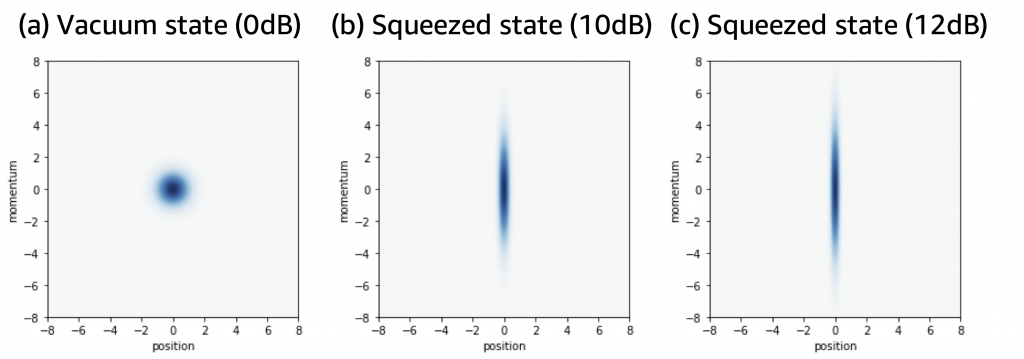Figure 2: Wigner functions of (a) a vacuum state (0 dB), (b) a 10 dB squeezed state in the position quadrature, (c) a 12 dB squeezed state in the position quadrature.

However, in error correction scenarios, it is important to address errors in both position and momentum axes simultaneously, and thus this simple way of noise squeezing does not quite work for error correction. So the key design principle behind the GKP qubits is to give up the precision of position and momentum measurements and to maintain simultaneity. Giving up the precision may not sound like a great idea because in error correction, precision is key. However, in GKP qubits, precision is dropped in a clever way. That is, you measure the position and momentum operators precisely but only modulo a certain spacing. Here, the term “modulo” is used in the context of modulo operations (division and remainder). Because of the modularity, a GKP state forms a lattice in the phase space (see Figures 3a and 3b). In other words, within the unit cell of the underlying lattice (see the dashed lines in Figure 3), the position and the momentum of the GKP state are precisely determined (for example, center of each unit cell), but the Heisenberg uncertainty principle is still respected, because we do not know in which unit cell the GKP state sits in.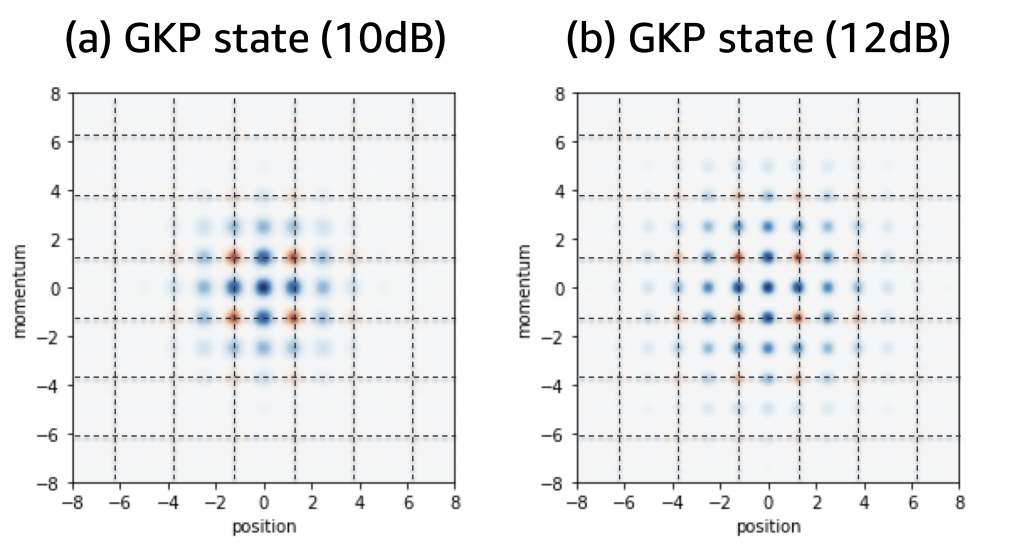Figure 3: Wigner functions of (a) a GKP state with 10 dB GKP squeezing (b) a GKP state with 12 dB GKP squeezing

Since both the position and momentum operators can be addressed simultaneously within the unit cell of the underlying GKP lattice, you can detect and correct any small shift errors to the GKP state as long as they are contained within the unit cell. On the other hand, you cannot correct a shift error that is large enough to map a unit cell to another unit cell. This is a small price to pay when giving up the absolute precision and instead going for a restricted precision, modulo a lattice spacing. However, this is not a big compromise, because smaller, correctable shift errors are much more likely to happen than larger, uncorrectable shift errors, such as physical shift errors due to photon loss. Furthermore, the thermal noise is also confined in a Gaussian distribution with a sharp peak (as shown Figure 3).

Another thing to keep in mind is that an ideal GKP state is infinitely squeezed and thus has an unbounded energy. As a result, an ideal GKP state cannot be realized in practice. In reality, GKP states are always finitely squeezed, meaning that each peak of the GKP state has a non-zero noise variance. As shown in Figure 3, a more highly squeezed GKP state (Figure 3b) has narrower and sharper peaks and at the same time occupies more space than a less squeezed GKP state (Figure 3a). Such a finite width of each peak in realistic GKP states is the main source of error when working with GKP qubits. However, as long as GKP states are sufficiently squeezed (for example, with a GKP squeezing higher than 10 dB), the width of each peak is much smaller than the lattice spacing. Hence, the shifts due to finite squeezing are well contained within the unit cell of the underlying GKP lattice most of the time.

While the shift errors due to finite squeezing is contained within the unit cell of the GKP lattice most of the time, it may still slip from time to time. Currently available GKP states have a GKP squeezing that ranges from 5.5 dB to 9.5 dB [see Flühmann et al., Nature 566, 513–517 (2019) and Campagne-Ibarcq et al., Nature 584, 368–372 (2020)]. Even with 9.5 dB GKP qubits, a large (hence uncorrectable) shift error may happen once in every hundred applications of a gate (such as the CNOT gate). So, currently available GKP qubits won’t be reliable enough to run quantum algorithms of practical importance, which require a very low logical error rate. Thus, to achieve such a low logical error rate, an extra layer of protection is needed. Similarly, as in the case of bare two-level qubits, such extra protection can be offered by the surface code. Hence, we investigate the combination of the GKP code with the surface code, i.e., the surface-GKP code. In particular, we explain how the extra information gathered from the GKP error correction can improve the performance of the outer code, or the surface code.

Recall that the ability to measure the position and momentum operators simultaneously modulo a lattice spacing provides the GKP qubits with the first layer of protection against shift errors. Say the relevant lattice spacing is s for the GKP qubits. Then, any shift errors of size smaller than s/2 can be correctly identified and countered (see the blue arrow in Figure 4). On the other hand, those of size larger than s/2 may lead to errors (see the red arrow in Figure 4). The outer code, the surface code, provides the second layer of protection by correcting such residual errors that are left uncorrected by the GKP shift correction.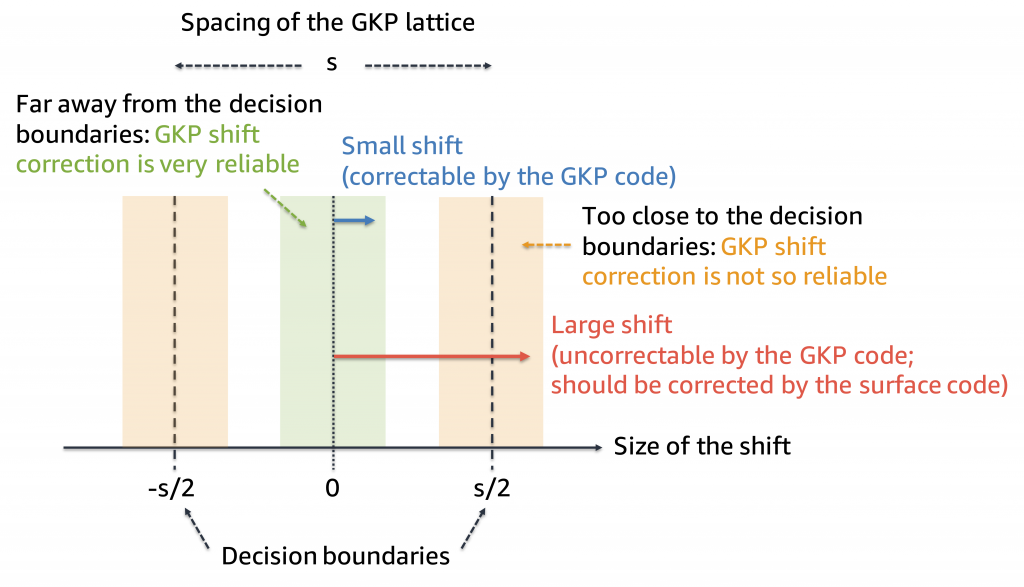Figure 4: Examples of correctable (blue arrow) and uncorrectable (red arrow) shifts and analog information based on the closeness to the decision boundaries: reliable (green shaded region) and unreliable (yellow shaded region) regions for the GKP shift correction.

Remarkably, as first noted in Fukui et al., Phys. Rev. Lett. 119, 180507 (2017), even when the GKP shift correction fails due to a large shift error, it leaves useful analog information that can be used to significantly boost the performance of the outer code. The key idea behind the analog information is that you can evaluate the reliability of the GKP shift correction by inspecting whether the shift correction occurred near the decision boundaries (at ±s/2; where the crossover between correctable and uncorrectable shift happens) or far away from the decision boundaries. It’s called analog information because the distance to the decision boundaries can take any continuous values, not discrete ones.

If the shift correction occurred far away from the decision boundaries (green region in Figure 4), you can safely assume that the GKP shift correction succeeded with high confidence. However, if the GKP shift correction happened near the decision boundaries (yellow region in Figure 4), even a small deviation can convert a correctable shift to an uncorrectable shift (and vice versa), so the GKP shift correction tends to be not quite reliable in this case. In most cases, an uncorrectable large shift typically falls into the yellow region where you know that the GKP shift correction should not be trusted too much (for example, see the red arrow in Figure 4). Thus, by passing this information to the next-level surface code error correction, you can help the surface-code decoder to identify more error-prone GKP qubits. This in turn lets the surface-code decoder make a more informed decision in correcting the residual errors that are left uncorrected by the GKP code. The access to such analog information is a unique feature of the GKP qubits, which is absent in bare two-level qubits.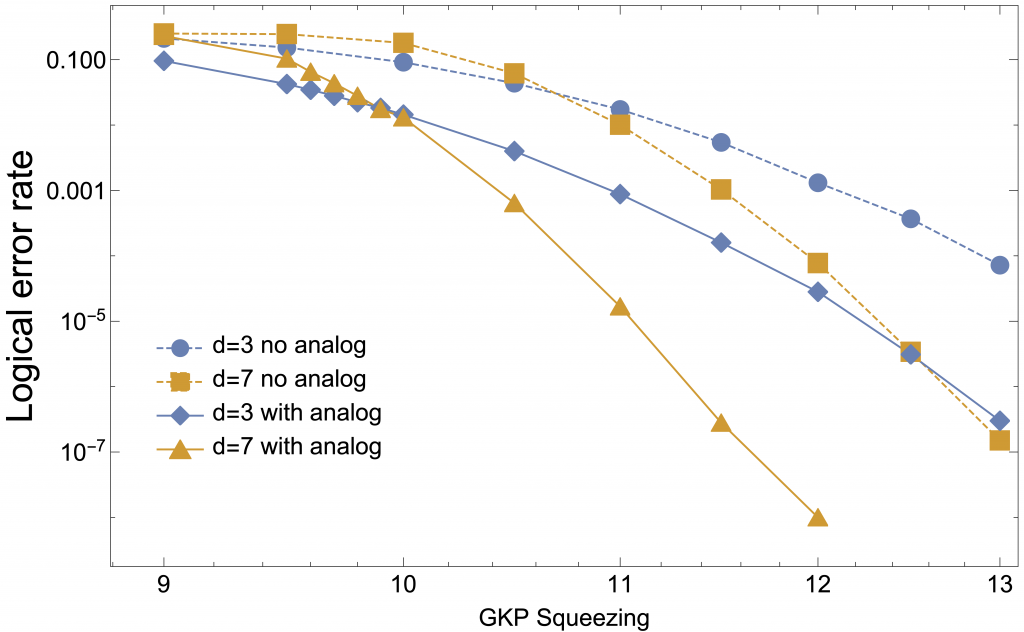## Surface-GKP code: hardware efficiency

To put our results into perspective, we compare the hardware resource overhead of the surface-GKP code approach with that of the traditional surface code approach using bare two-level qubits. When doing so, it is important to note that there’s an upfront cost of using GKP qubits. The reason is that GKP qubits need to be error corrected themselves, and the analog information should be gathered along the way. Thus, each GKP qubit requires three oscillator modes and one ancilla qubit (in our proposed realization of the GKP qubit in our paper). Therefore, at least four physical elements are needed to implement just a single GKP qubit. However, don’t forget that all these extra resources have a purpose: to gather useful analog information that is very helpful to reduce error rates. Also, the oscillator modes should not be treated on the same footing as the qubits since small phononic modes or multi-mode cavities can implement multiple oscillator modes in a hardware-efficient way.

One of the key conclusions of our research in this paper is that despite the extra overhead associated with implementing the GKP error correction (four physical elements per each GKP qubit), the analog information gathered from the GKP error correction is extremely useful, and thus this extra overhead pays off. To make the discussion concrete, assume that we have access to 12-dB GKP states. The error rate of the CNOT gate (to be used in the surface code error correction) between two 12-dB GKP qubits is given by 0.36%. The 0.36% error rate is comparable to what can be achieved with the state-of-the-art two-level qubit technologies. As summarized in Table 1, with such a two-qubit error rate, we estimate that the traditional surface code approach requires 1457 bare two-level qubits to achieve a logical error rate below 10−7 since a very large surface code distance of 27 is required to get there. On the other hand, the surface-GKP code approach only requires a distance-7 outer surface code and hence 97 GKP qubits. As a result, even with the upfront cost associated with implementing the GKP shift correction, the surface-GKP code requires only 291 oscillator modes and 97 qubits, as opposed to 1457 bare two-level qubits. Again, such a resource overhead reduction is possible through the help of extra analog information gathered from the GKP shift correction.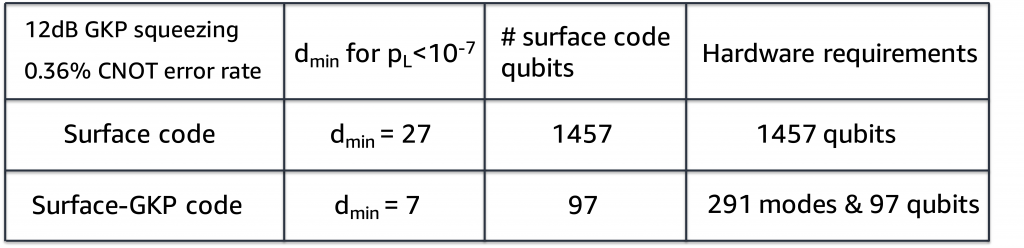Table 1: Resource overhead comparison between the surface-GKP code approach and the traditional surface-code approach. This shows the surface-GKP code approach with 12dB GKP states (giving a CNOT error rate of 0.36%) and the traditional surface-code approach with bare two-level qubits (superconducting qubits or trapped-ion qubits) at an equivalent CNOT gate error rate of 0.36%.

## Conclusion and outlook

Our results indicate that GKP qubits with a GKP squeezing around 12 dB are very promising for achieving a low logical error rate in a hardware-efficient manner. On the other hand, the highest GKP squeezing that has been experimentally achieved so far is about 9.5 dB, so more work is needed to increase the GKP squeezing and make GKP qubits functional. Recently, however, better GKP state preparation schemes have been theoretically proposed [Royer et al., Phys. Rev. Lett. 125, 260509 (2020)], so there is a good chance of improving upon the previously achieved GKP squeezing of 9.5 dB. Another key result of our paper is that the 12-dB GKP squeezing can be experimentally realized, in principle, by using the improved GKP state preparation schemes. This can be done with a few modifications to make them robust against dominant experimental imperfections, and if you assume realistic experimental parameters. Thus, thanks to the various community efforts on GKP qubits, both on the experimental and theoretical sides (including but not limited to Gottesman 2001, Fukui 2017, Flühmann 2019, Campagne-Ibarcq 2020, Royer 2020, and our work), GKP qubits are becoming an even more attractive option for achieving the low logical error rates needed for quantum computers to run practical quantum algorithms.

There is much more work to be done as an industry to bring these ideas to fruition in a fault-tolerant quantum computer, and new technologies have opened up many exciting opportunities. If you’d like to join the quantum computing mission at AWS, check out our open jobs. We’re hiring in research science, software, algorithms, and hardware development. And if you want to use current quantum computers for your own research in quantum algorithms and hardware, check out Amazon Braket and the AWS Cloud Credit for Research program.